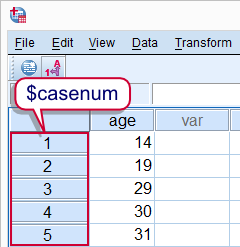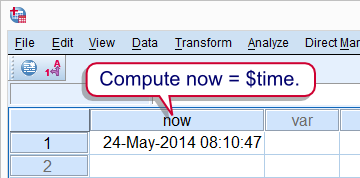# SPSS tutorials

BASICS DATA ANALYSIS T-TEST ANOVA CHI-SQUARE TEST

# SPSS System Variables

## Summary

SPSS uses a handful of hidden variables for keeping track of things. These so called system variables have names starting with "\$" and can be useful in some cases. The most important one is \$casenum, the number shown next to each case in the data editor window.

## SPSS \$casenumSPSS \$casenum

In the left outline of the data editor window (under Data View) we see a number next to each case. This number is internally known as `\$casenum`. To some extent we can use `\$casenum` in normal SPSS commands. We can also convert it into a regular variable. This can be used to create a unique row id if this is absent. The syntax below demonstrates this.

## SPSS Syntax Example 1

*1. Create test data.

data list free/age.
begin data
14 19 29 30 31
end data.

*2. Use \$casenum in regular command.

compute first_case = \$casenum eq 1.
exe.

*3. Convert \$casenum to normal variable.

compute id = \$casenum.
exe.

## SPSS Select If and \$casenum

Using `SELECT IF` with `\$casenum` can be tricky. This is because SPSS uses `\$casenum` to keep track of cases during procedures. If cases are deleted during a procedure, their `\$casenum` may shift to a different case. Like so, `SELECT IF \$casenum` GT 1. will delete all cases in the data. What happens, is this

1. SPSS starts at the first case. Since its `\$casenum` is not greater than one, it's deleted.
2. The second case now shifts up one row and is thus assigned `\$casenum` 1.
3. Since the second case's `\$casenum` is not greater than one (anymore), it's deleted as well.
4. Now the third case shifts up and gets `\$casenum` 1. And so on.

## SPSS \$time

A second system variable that may sometimes be useful is \$time. This is hold the current date and time. As with \$casenum, it can be used straight away, for example in `DATEDIFF`. Alternatively, its value can be passed into a regular variable as demonstrated by the final syntax example.SPSS \$time

## SPSS Syntax Example 2

*1. Create single case test data.

data list free/now.
begin data
0
end data.

*2. Compute now = current date and time.

compute now = \$TIME.
exe.

*3. Display now as datetime variable.

formats now(datetime22).

## Final Note

SPSS uses more system variables than `\$casenum` and `\$time`. Consult the command syntax reference for a complete overview.

# Let me know what you think!

*Required field. Your comment will show up after approval from a moderator.

# This tutorial has 1 comment!

•### By Natalka Patsiurko on January 17th, 2016

Hi Ruben,
still using your tutorials and still find them very useful. In this one I again found an answer to my question. Just wanted to say thanks!